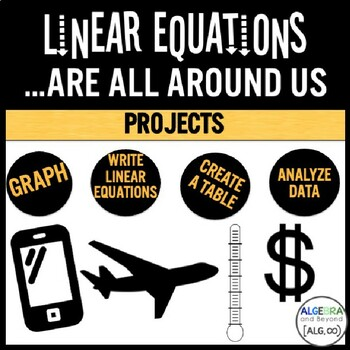# Real World Linear Equations | Project Based Learning | Distance LearningGrade Levels
7th - 9th
Subjects
Standards
Resource Type
Formats Included
• Zip
Pages
5 pages

#### Also included in

1. This bundle is a great addition to any Algebra curriculum! It includes activities from the following product lines:Name That Function: activities that help students critically think about graphs and their characteristics.Battle My Math Ship: a fun activity to keep students engaged in practicing thei
\$100.00
\$162.50
Save \$62.50

### Description

Linear equations in the real world! This resource includes FOUR different projects (100% editable) from basic to advanced levels of Pre-Algebra and Algebra.

The real-life topics:

• Cell phone plans

• Exchange rates

• Temperature conversions

• Frequent flyer miles

Linear representations:

• Equations

• Tables

• Graphs

• Words

TWO EDITABLE rubrics included to fit your classroom needs!

Check out the preview to see what skills are covered.

∞∞∞∞∞∞∞∞∞∞∞∞∞∞∞∞∞∞∞∞∞∞∞∞∞∞∞∞∞∞∞∞∞∞∞∞∞∞∞∞∞∞∞∞∞∞∞

Learn more about Algebra and Beyond's resources:

© Algebra and Beyond 2015

This product is intended for personal use in one classroom only. For use in multiple classrooms, please purchase additional licenses.

Total Pages
5 pages
Answer Key
Included with rubric
Teaching Duration
2 days
Report this Resource to TpT
Reported resources will be reviewed by our team. Report this resource to let us know if this resource violates TpT’s content guidelines.

### Standards

to see state-specific standards (only available in the US).
Describe qualitatively the functional relationship between two quantities by analyzing a graph (e.g., where the function is increasing or decreasing, linear or nonlinear). Sketch a graph that exhibits the qualitative features of a function that has been described verbally.
Construct a function to model a linear relationship between two quantities. Determine the rate of change and initial value of the function from a description of a relationship or from two (𝘹, 𝘺) values, including reading these from a table or from a graph. Interpret the rate of change and initial value of a linear function in terms of the situation it models, and in terms of its graph or a table of values.
Interpret the equation 𝘺 = 𝘮𝘹 + 𝘣 as defining a linear function, whose graph is a straight line; give examples of functions that are not linear. For example, the function 𝘈 = 𝑠² giving the area of a square as a function of its side length is not linear because its graph contains the points (1,1), (2,4) and (3,9), which are not on a straight line.
Compare properties of two functions each represented in a different way (algebraically, graphically, numerically in tables, or by verbal descriptions). For example, given a linear function represented by a table of values and a linear function represented by an algebraic expression, determine which function has the greater rate of change.
Understand that a function is a rule that assigns to each input exactly one output. The graph of a function is the set of ordered pairs consisting of an input and the corresponding output.

### Questions & Answers

Teachers Pay Teachers is an online marketplace where teachers buy and sell original educational materials.

More About Us